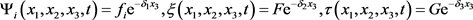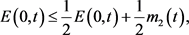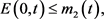﻿ 一类热扩散方程组的空间衰减估计

# 一类热扩散方程组的空间衰减估计Spatial Decay Estimates for a Class of Thermo-Diffusion Equations

Abstract: In this paper, we study the spatial decay estimates for a class of thermo-diffusion equations. Using the technique of a first-order differential inequality, the exponential decay estimates for the linear differential equations of thermodiffusion in a semi-infinite pipe were established. To make the estimate explicit, the bound for the total energy was also derived.

1. 前言

${R}_{z}=\left\{\left({x}_{1},{x}_{2},{x}_{3}\right)|\left({x}_{1},{x}_{2}\right)\in D,{x}_{3}>z\ge 0\right\},$

${D}_{z}=\left\{\left({x}_{1},{x}_{2},{x}_{3}\right)|\left({x}_{1},{x}_{2}\right)\in D,{x}_{3}=z\ge 0\right\},$

$\rho {\stackrel{¨}{\mu }}_{i}-v\Delta {u}_{i}-\left(\lambda +v\right){u}_{j,ji}+{\gamma }_{1}{T}_{,i}+{\gamma }_{2}{C}_{,i}=0,\text{\hspace{0.17em}}\text{\hspace{0.17em}}\text{\hspace{0.17em}}R×\left\{t\ge 0\right\},$ (1.1)

$c\stackrel{˙}{T}-K\Delta T+{\gamma }_{1}{\stackrel{˙}{u}}_{i,i}+d\stackrel{˙}{C}=0,\text{\hspace{0.17em}}\text{\hspace{0.17em}}\text{\hspace{0.17em}}R×\left\{t\ge 0\right\},$ (1.2)

$n\stackrel{˙}{C}-M\Delta C+{\gamma }_{2}{\stackrel{˙}{u}}_{i,i}+d\stackrel{˙}{T}=0,\text{\hspace{0.17em}}\text{\hspace{0.17em}}\text{in}\text{\hspace{0.17em}}R×\left\{t\ge 0\right\},$ (1.3)

${u}_{i}=0,T=C=0\text{\hspace{0.17em}}\text{\hspace{0.17em}}\text{\hspace{0.17em}}\text{on}\text{\hspace{0.17em}}\partial D×\left\{t\ge 0\right\},$ (1.4)

${u}_{i}=\stackrel{˙}{u}=0,T=C=0\text{\hspace{0.17em}}\text{\hspace{0.17em}}\text{\hspace{0.17em}}\text{in}\text{\hspace{0.17em}}R×\left\{t=0\right\}\text{.}$ (1.5)

${u}_{i}={f}_{i}\left({x}_{1},{x}_{2},t\right),T=F\left({x}_{1},{x}_{2},t\right),C=G\left({x}_{1},{x}_{2},t\right)\text{\hspace{0.17em}}\text{\hspace{0.17em}}\text{\hspace{0.17em}}\text{on}\text{\hspace{0.17em}}{D}_{0}×\left\{t\ge 0\right\},$ (1.6)

${u}_{i},{u}_{i,j},T,{T}_{i},C,{C}_{,i},p=o\left({x}_{3}^{-1}\right){x}_{1},{x}_{2},t\text{\hspace{0.17em}}\text{\hspace{0.17em}}\text{\hspace{0.17em}}\text{as}\text{\hspace{0.17em}}{x}_{3}\to \infty \text{.}$ (1.7)

$cn-{d}^{2}>0$ (1.8)

${\int }_{D}{w}_{,a}{w}_{,a}\text{d}A\ge {\lambda }_{1}{\int }_{D}{w}^{2}\text{d}x,$ (1.9)

$\Delta \phi +\lambda \phi =0\text{\hspace{0.17em}}\text{\hspace{0.17em}}\text{\hspace{0.17em}}\text{in}\text{\hspace{0.17em}}D,$

$\phi =0\text{\hspace{0.17em}}\text{\hspace{0.17em}}\text{\hspace{0.17em}}\text{on}\text{\hspace{0.17em}}\partial D$

${u}_{i,j}=\frac{\partial {u}_{i}}{\partial {x}_{i}},{\phi }_{\alpha ,\alpha }={\sum }_{\alpha =1}^{2}\frac{\partial {\phi }_{\alpha }}{\partial {x}_{\alpha }},{\stackrel{˙}{u}}_{i}=\frac{\partial {u}_{i}}{{\partial }_{t}}.$

2. 能量衰减

$\begin{array}{c}0={\int }_{0}^{t}{\int }_{{R}_{z}}\left[\rho {\stackrel{¨}{u}}_{i}-v\Delta {u}_{i}-\left(\lambda +v\right){u}_{j,ji}+{\gamma }_{1}{T}_{,i}+{\gamma }_{2}{C}_{,i}\right]{\stackrel{˙}{u}}_{i}\text{d}x\text{d}\eta \\ =\frac{1}{2}\rho {\int }_{{R}_{z}}{\stackrel{˙}{u}}_{i}{\stackrel{˙}{u}}_{i}\text{d}x+v{\int }_{0}^{t}{\int }_{{D}_{z}}{u}_{i,3}{\stackrel{˙}{u}}_{i}\text{d}A\text{d}\eta +\frac{v}{2}{\int }_{{R}_{z}}{u}_{i,j}{u}_{i,j}\text{d}x+\left(\lambda +v\right){\int }_{0}^{t}{\int }_{{D}_{z}}{u}_{j,j}{\stackrel{˙}{u}}_{3}\text{d}A\text{d}\eta \\ \text{\hspace{0.17em}}\text{\hspace{0.17em}}+\frac{\lambda +v}{2}{\int }_{{R}_{z}}{u}_{i,i}^{2}\text{d}x+{\gamma }_{1}{\int }_{0}^{t}{\int }_{{R}_{z}}{T}_{,i}{\stackrel{˙}{u}}_{i}\text{d}x\text{d}\eta +{\gamma }_{2}{\int }_{0}^{t}{\int }_{{R}_{z}}{C}_{,i}{\stackrel{˙}{u}}_{i}\text{d}x\text{d}\eta \text{\hspace{0.17em}}.\end{array}$ (2.1)

$\begin{array}{c}0={\int }_{0}^{t}{\int }_{{R}_{z}}\left[c\stackrel{˙}{T}-K\Delta T+{\gamma }_{1}{\stackrel{˙}{u}}_{i,i}+d\stackrel{˙}{C}\right]T\text{d}x\text{d}\eta \\ =\frac{c}{2}{\int }_{{R}_{z}}{{T}^{2}\text{d}x|}_{\eta =t}+K{\int }_{0}^{t}{\int }_{{D}_{z}}{T}_{,3}T\text{d}A\text{d}\eta +K{\int }_{0}^{t}{\int }_{{R}_{z}}{T}_{,j}{T}_{,j}\text{d}x\text{d}\eta \\ \text{\hspace{0.17em}}\text{\hspace{0.17em}}-{\gamma }_{1}{\int }_{0}^{t}{\int }_{{D}_{z}}T{\stackrel{˙}{u}}_{3}\text{d}A\text{d}\eta -{\gamma }_{1}{\int }_{0}^{t}{\int }_{{R}_{z}}{T}_{,i}{\stackrel{˙}{u}}_{i}\text{d}x\text{d}\eta +d{\int }_{0}^{t}{\int }_{{R}_{z}}C\stackrel{˙}{T}\text{d}x\text{d}\eta \text{\hspace{0.17em}}\text{.}\end{array}$ (2.2)

$\begin{array}{c}0={\int }_{0}^{t}{\int }_{{R}_{z}}\left[n\stackrel{˙}{C}-M\Delta C+{\gamma }_{2}{\stackrel{˙}{u}}_{i,i}+d\stackrel{˙}{T}\right]C\text{d}x\text{d}\eta \\ =\frac{n}{2}{\int }_{{R}_{z}}{{C}^{2}\text{d}x|}_{\eta =t}+M{\int }_{0}^{t}{\int }_{{D}_{z}}{C}_{,3}C\text{d}A\text{d}\eta +M{\int }_{0}^{t}{\int }_{{R}_{z}}{C}_{,j}{C}_{,j}\text{d}x\text{d}\eta \\ \text{\hspace{0.17em}}\text{\hspace{0.17em}}-{\gamma }_{2}{\int }_{0}^{t}{\int }_{{D}_{z}}C{\stackrel{˙}{u}}_{3}\text{d}A\text{d}\eta -{\gamma }_{2}{\int }_{0}^{t}{\int }_{{R}_{z}}{C}_{,i}{\stackrel{˙}{u}}_{i}\text{d}x\text{d}\eta +d{\int }_{0}^{t}{\int }_{{R}_{z}}C\stackrel{˙}{T}\text{d}x\text{d}\eta \end{array}$ (2.3)

$\begin{array}{c}E\left(z,t\right)=\frac{1}{2}\rho {\int }_{{R}_{z}}{\stackrel{˙}{u}}_{i}{\stackrel{˙}{u}}_{i}\text{d}x+\frac{v}{2}{\int }_{{R}_{z}}{u}_{i,j}{u}_{i,j}\text{d}x+\frac{\lambda +v}{2}{\int }_{{R}_{z}}{u}_{i,i}^{2}\text{d}x+K{\int }_{0}^{t}{\int }_{{R}_{z}}{T}_{,j}{T}_{,j}\text{d}x\text{d}\eta \\ \text{\hspace{0.17em}}\text{\hspace{0.17em}}+M{\int }_{0}^{t}{\int }_{{R}_{z}}{C}_{,j}{C}_{,j}\text{d}x\text{d}\eta +{\int }_{{R}_{z}}\left[\frac{c}{2}{T}^{2}+dCT+\frac{n}{2}{C}^{2}\right]\text{d}x\end{array}$ (2.4)

$\begin{array}{c}-\frac{\partial E\left(z,t\right)}{\partial z}=\frac{1}{2}\rho {\int }_{{D}_{z}}{\stackrel{˙}{u}}_{i}{\stackrel{˙}{u}}_{i}\text{d}A+\frac{v}{2}{\int }_{{D}_{z}}{u}_{i,j}{u}_{i,j}\text{d}A+\frac{\lambda +v}{2}{\int }_{{D}_{z}}{u}_{i,i}^{2}\text{d}A+K{\int }_{0}^{t}{\int }_{{D}_{z}}{T}_{,j}{T}_{,j}\text{d}A\text{d}\eta \\ \text{\hspace{0.17em}}\text{\hspace{0.17em}}+M{\int }_{0}^{t}{\int }_{{D}_{z}}{C}_{,j}{C}_{,j}\text{d}A\text{d}\eta +{\int }_{{D}_{z}}\left[\frac{c}{2}{T}^{2}+dCT+\frac{n}{2}{C}^{2}\right]\text{d}A\text{\hspace{0.17em}}\text{.}\end{array}$ (2.5)

$\begin{array}{c}E\left(z,t\right)=-v{\int }_{0}^{t}{\int }_{{D}_{z}}{u}_{i,3}{\stackrel{˙}{u}}_{i}\text{d}A\text{d}\eta -\left(\lambda +v\right){\int }_{0}^{t}{\int }_{{D}_{z}}{u}_{j,j}{\stackrel{˙}{u}}_{3}\text{d}A\text{d}\eta +{\gamma }_{1}{\int }_{0}^{t}{\int }_{{D}_{z}}T{\stackrel{˙}{u}}_{3}\text{d}A\text{d}\eta \\ \text{\hspace{0.17em}}\text{\hspace{0.17em}}+{\gamma }_{2}{\int }_{0}^{t}{\int }_{{D}_{z}}C{\stackrel{˙}{u}}_{3}\text{d}A\text{d}\eta -K{\int }_{0}^{t}{\int }_{{D}_{z}}{T}_{,3}T\text{d}A\text{d}\eta -M{\int }_{0}^{t}{\int }_{{D}_{z}}{C}_{,3}C\text{d}A\text{d}\eta \text{\hspace{0.17em}}.\end{array}$ (2.6)

$\begin{array}{c}v{\int }_{0}^{t}{\int }_{{D}_{z}}{u}_{i,3}{\stackrel{˙}{u}}_{i}\text{d}A\text{d}\eta \le v{\left({\int }_{0}^{t}{\int }_{{D}_{z}}{\stackrel{˙}{u}}_{i}{\stackrel{˙}{u}}_{i}\text{d}A\text{d}\eta \right)}^{\frac{1}{2}}{\left({\int }_{0}^{t}{\int }_{{D}_{z}}{u}_{i,3}{u}_{i,3}\text{d}A\text{d}\eta \right)}^{\frac{1}{2}}\\ \le v{\left({\int }_{0}^{t}{\int }_{{D}_{z}}{\stackrel{˙}{u}}_{i}{\stackrel{˙}{u}}_{i}\text{d}A\text{d}\eta \right)}^{\frac{1}{2}}{\left({\int }_{0}^{t}{\int }_{{D}_{z}}{u}_{i,3}{u}_{i,3}\text{d}A\text{d}\eta \right)}^{\frac{1}{2}}\\ \le \frac{\sqrt{v}t}{\sqrt{\rho }}\left(-\frac{\partial E\left(z,t\right)}{\partial z}\right),\end{array}$ (2.7)

$\begin{array}{c}\left(\lambda +v\right){\int }_{0}^{t}{\int }_{{D}_{z}}{u}_{j,j}\stackrel{˙}{u}\text{d}A\text{d}\eta \le \left(\lambda +v\right){\left({\int }_{0}^{t}{\int }_{{D}_{z}}{\stackrel{˙}{u}}_{i}{\stackrel{˙}{u}}_{i}\text{d}A\text{d}\eta \right)}^{\frac{1}{2}}{\left({\int }_{0}^{t}{\int }_{{D}_{z}}{u}_{j,j}^{2}\text{d}A\text{d}\eta \right)}^{\frac{1}{2}}\\ \begin{array}{c}\le \frac{\sqrt{\lambda +vt}}{\sqrt{\rho }}\left(-\frac{\partial E\left(z,t\right)}{\partial z}\right),\end{array}\end{array}$ (2.8)

$\begin{array}{l}{\gamma }_{1}{\int }_{0}^{t}{\int }_{{D}_{z}}T{\stackrel{˙}{u}}_{3}\text{d}A\text{d}\eta +{\gamma }_{2}{\int }_{0}^{t}{\int }_{{D}_{z}}C{\stackrel{˙}{u}}_{3}\text{d}A\text{d}\eta \\ \le {\gamma }_{1}{\left({\int }_{0}^{t}{\int }_{{D}_{z}}{T}^{2}\text{d}A\text{d}\eta \right)}^{\frac{1}{2}}{\left({\int }_{0}^{t}{\int }_{{D}_{z}}{\stackrel{˙}{u}}_{3}^{2}\text{d}A\text{d}\eta \right)}^{\frac{1}{2}}+{\gamma }_{2}{\left({\int }_{0}^{t}{\int }_{{D}_{z}}{C}^{2}\text{d}A\text{d}\eta \right)}^{\frac{1}{2}}{\left({\int }_{0}^{t}{\int }_{{D}_{z}}{\stackrel{˙}{u}}_{3}^{2}\text{d}A\text{d}\eta \right)}^{\frac{1}{2}}\\ \le \frac{{\gamma }_{1}}{\sqrt{{\lambda }_{1}}}{\left({\int }_{0}^{t}{\int }_{{D}_{z}}{T}_{,\alpha }{T}_{,\alpha }\text{d}A\text{d}\eta \right)}^{\frac{1}{2}}{\left({\int }_{0}^{t}{\int }_{{D}_{z}}{\stackrel{˙}{u}}_{3}^{2}\text{d}A\text{d}\eta \right)}^{\frac{1}{2}}+\frac{{\gamma }_{2}}{\sqrt{{\lambda }_{1}}}{\left({\int }_{0}^{t}{\int }_{{D}_{z}}{C}_{,\alpha }{C}_{,\alpha }\text{d}A\text{d}\eta \right)}^{\frac{1}{2}}{\left({\int }_{0}^{t}{\int }_{{D}_{z}}{\stackrel{˙}{u}}_{3}^{2}\text{d}A\text{d}\eta \right)}^{\frac{1}{2}}\\ \le \left[\frac{{\gamma }_{1}\sqrt{t}}{2\sqrt{\rho {\lambda }_{1}K}}+\frac{{\gamma }_{2}\sqrt{t}}{2\sqrt{\rho {\lambda }_{1}M}}\right]\left(-\frac{\partial E\left(z,t\right)}{\partial z}\right).\end{array}$ (2.9)

$\begin{array}{l}-K{\int }_{0}^{t}{\int }_{{D}_{z}}{T}_{,3}T\text{d}A\text{d}\eta -M{\int }_{0}^{t}{\int }_{{D}_{z}}{C}_{,3}C\text{d}A\text{d}\eta \\ \le K{\left({\int }_{0}^{t}{\int }_{{D}_{z}}{T}_{,3}^{2}\text{d}A\text{d}\eta \right)}^{\frac{1}{2}}{\left({\int }_{0}^{t}{\int }_{{D}_{z}}{T}^{2}\text{d}A\text{d}\eta \right)}^{\frac{1}{2}}+M{\left({\int }_{0}^{t}{\int }_{{D}_{z}}{C}_{,3}^{2}\text{d}A\text{d}\eta \right)}^{\frac{1}{2}}{\left({\int }_{0}^{t}{\int }_{{D}_{z}}{C}^{2}\text{d}A\text{d}\eta \right)}^{\frac{1}{2}}\\ \le \frac{K}{\sqrt{{\lambda }_{1}}}{\left({\int }_{0}^{t}{\int }_{{D}_{z}}{T}_{,3}^{2}\text{d}A\text{d}\eta \right)}^{\frac{1}{2}}{\left({\int }_{0}^{t}{\int }_{{D}_{z}}{T}_{,\alpha }{T}_{,\alpha }\text{d}A\text{d}\eta \right)}^{\frac{1}{2}}+\frac{M}{\sqrt{{\lambda }_{1}}}{\left({\int }_{0}^{t}{\int }_{{D}_{z}}{C}_{,3}^{2}\text{d}A\text{d}\eta \right)}^{\frac{1}{2}}{\left({\int }_{0}^{t}{\int }_{{D}_{z}}{C}_{,\alpha }{C}_{,\alpha }\text{d}A\text{d}\eta \right)}^{\frac{1}{2}}\\ \begin{array}{c}\le \frac{1}{2\sqrt{{\lambda }_{1}}}\left(-\frac{\partial E\left(z,t\right)}{\partial z}\right).\end{array}\end{array}$ (2.10)

$E\left(z,t\right)\le {m}_{1}\left(t\right)\left(-\frac{\partial E\left(z,t\right)}{\partial z}\right),$ (2.11)

${m}_{1}\left(t\right)=\frac{\sqrt{v}t}{\sqrt{\rho }}+\frac{\sqrt{\lambda +vt}}{\sqrt{\rho }}+\frac{{\gamma }_{1}\sqrt{t}}{2\sqrt{\rho {\lambda }_{1}K}}+\frac{{\gamma }_{2}\sqrt{t}}{2\sqrt{\rho {\lambda }_{1}M}}+\frac{1}{2\sqrt{{\lambda }_{1}}}$ (2.12)

$E\left(z,t\right)\le E\left(0,t\right){\text{e}}^{-\frac{1}{{m}_{1}\left(t\right)}z}.$ (2.13)

3. 总能量E(0, t)的边界

$\begin{array}{c}E\left(0,t\right)=\frac{1}{2}\rho {\int }_{R}{\stackrel{˙}{u}}_{i}{\stackrel{˙}{u}}_{i}\text{d}x+\frac{v}{2}{\int }_{R}{u}_{i,j}{u}_{i,j}\text{d}x+\frac{\lambda +v}{2}{\int }_{R}{u}_{i,i}^{2}\text{d}x+K{\int }_{0}^{t}{\int }_{R}{T}_{,j}{T}_{,j}\text{d}x\text{d}\eta \\ \text{\hspace{0.17em}}\text{\hspace{0.17em}}+M{\int }_{0}^{t}{\int }_{R}{C}_{,j}{C}_{,j}\text{d}x\text{d}\eta +{\int }_{R}\left[\frac{c}{2}{T}^{2}+dCT+\frac{n}{2}{C}^{2}\right]\text{d}x\\ =-v{\int }_{0}^{t}{\int }_{{D}_{0}}{u}_{i,3}{\stackrel{˙}{u}}_{i}\text{d}A\text{d}\eta -\left(\lambda +v\right){\int }_{0}^{t}{\int }_{{D}_{0}}{u}_{j,j}{\stackrel{˙}{u}}_{3}\text{d}A\text{d}\eta +{\gamma }_{1}{\int }_{0}^{t}{\int }_{{D}_{0}}T{\stackrel{˙}{u}}_{3}\text{d}A\text{d}\eta \\ \text{\hspace{0.17em}}\text{\hspace{0.17em}}+{\gamma }_{2}{\int }_{0}^{t}{\int }_{{D}_{0}}C{\stackrel{˙}{u}}_{3}\text{d}A\text{d}\eta -K{\int }_{0}^{t}{\int }_{{D}_{0}}{T}_{,3}T\text{d}A\text{d}\eta -M{\int }_{0}^{t}{\int }_{{D}_{0}}{C}_{,3}C\text{d}A\text{d}\eta \text{\hspace{0.17em}}.\end{array}$ (3.1)(3.2)(3.3)(3.4)(3.5)(3.6)(3.7)(3.8)

 Nowacki, W. (1971) Certain Problem of Thermo-Diffusion in Solids. Archives of Mechanics, 23, 731-754.

 Fichera, G. (1974) Uniqueness, Existence and Estimates of Solution in the Dynamical Problem of Thermodiffusion in an Elastic Solid. Archives of Mechanics, 26, 903-920.

 Podstrigach, Y.S. (1961) Differential Equations of the Problem of Thermodiffusion in an Isotropic Deformable Solid. Dopovidi Akademi? nauk Ukra?ns ko? RSR, 2, 169-172. (In Ukrainian)

 Szymaniec, A. (2010) Lp-Lq Time Decay Estimates for the Solution of the Linear Partial Differential Equations of Thermodiffusion. Applicationes Mathematicae, 37, 143-170.
https://doi.org/10.4064/am37-2-2

 Kaloni, P.N. and Qin, Y. (1998) Spatial Decay Estimates for Flow in the Brinkman-Forchheimer Model. Quarterly of Applied Mathematics, 56, 71-87.
https://doi.org/10.1090/qam/1604880

 Horgan, C.O. and Wheeler, L.T. (1978) Spatial Decay Estimates for the Navier-Stokes Equations with Application to the Problem of Entry Flow. SIAM Journal on Applied Mathematics, 35, 97-116.
https://doi.org/10.1137/0135008

 Payne, L.E. and Song, J.C. (1997) Spatial Decay Estimates for the Brinkman and Darcy Flows in a Semi-Infinite Cylinder. Continuum Mechanics and Thermodynamics, 9, 175-190.
https://doi.org/10.1007/s001610050064

 Ames, K.A. and Song, J.C. (2006) Decay Bounds for Magnetohydrodynamic Geophysical Flow. Nonlinear Analysis, 65, 1318-1333.
https://doi.org/10.1016/j.na.2005.10.013

 Ames, K.A. and Payne, L.E. (1989) Decay Estimates in Steady Pipe Flow. SIAM Journal on Mathematical Analysis, 20, 789-815.
https://doi.org/10.1137/0520056

 Horgan, C.O. (1996) Recent Developments Concerning Saint-Venant’s Principle: A Second Update. Applied Mechanics Reviews, 49, 101-111.
https://doi.org/10.1115/1.3101961

 Lin, C. (1992) Spatial Decay Estimates and Energy Bounds for the Stokes Flow Equation. Stability & Applied Analysis of Continuous Media, 2, 249-264.

 Lin, C. and Payne, L.E. (2004) Spatial Decay Bounds in the Channel Flow of an Incompressible Viscous Fluid. Mathematical Models and Methods in Applied Sciences, 14, 795-818.
https://doi.org/10.1142/S0218202504003453

 Song, J.C. (2003) Improved Decay Estimates in Time-Dependent Stokes Flow. SIAM Journal on Mathematical Analysis, 288, 505-517.
https://doi.org/10.1016/j.jmaa.2003.09.007

 Payne, L.E. (1967) Isopermetric Inequalities and Their Applications. SIAM Review, 9, 453-488.
https://doi.org/10.1137/1009070

 Polya, G. and Szego, G. (1951) Isopermetric Inequalities in Mathematical Physics. Annals of Mathematics Studies Vol. 27, Princeton University Press, Princeton.

Top